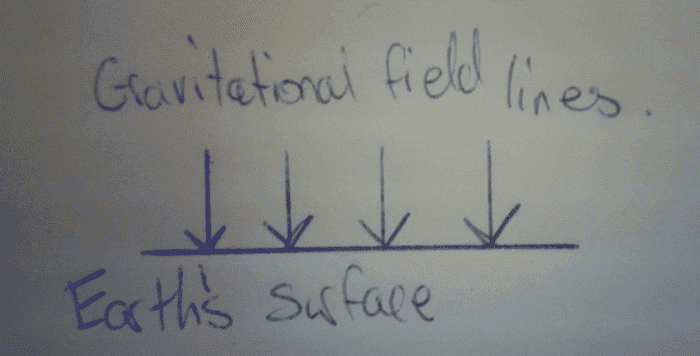# Gravitational fields

## Homework Statement

Need someone to confirm if I answered correctly. Thanks for your support :)

A) Draw the pattern of the gravitational flux passing through a horizontal surface A, of area 1m² close to the Earth's surface.
B) What is meant by the phrase "Gravitational potential at a point in a gravitational field"?
C) Explain why the surface A is an equipotential surface.
D) If a surface B, situated 2m directly below A and parallel to A, is taken to be at zero gravitational potential, what is the potential of surface A? Take the acceleration of free fall at the Earth's surface to be 10m/s²
E) If a body is allowed to fall from surface A to surface B along a smooth, curved path, what will be its velocity on reaching surface B?

## The Attempt at a Solution

A)B) The gravitational potential of a point in a gravitational field is the work done per unit mass by the pull of gravity to bring a body from infinity to that point
C) Since surface A has a uniform gravitational field rather than a radial, the gravitational field strength is a constant within surface A. That is called equipotential.
D) Knowing the Gravitational Constant: G = 6.674X10-11 m^3/kg/s^2
Making Mass subject = gr²/G = 6x1011kg
V=-GM/r= -(6.67x10-11*6x1011)/2
V= 20.01J
E) V=√2GM/r = √(6.67x10-11*6x1011*2)/2= 6.33m/s

Last edited: